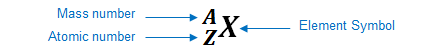# Problem: Choose the correct definition of an atom's atomic number.A. An atom's atomic number is the number of neutrons it has in its nucleus.B. An atom's atomic number is the number of particles it has in its nucleus.C. An atom's atomic number is the number of protons it has in its nucleus.D. An atom's atomic number is the number of electrons it has in its nucleus.

###### FREE Expert Solution

We’re being asked to choose the correct definition of an atom's atomic number.

An element can be represented as:Atomic number (Z):

86% (238 ratings)###### Problem Details

Choose the correct definition of an atom's atomic number.

A. An atom's atomic number is the number of neutrons it has in its nucleus.

B. An atom's atomic number is the number of particles it has in its nucleus.

C. An atom's atomic number is the number of protons it has in its nucleus.

D. An atom's atomic number is the number of electrons it has in its nucleus.

Frequently Asked Questions

What scientific concept do you need to know in order to solve this problem?

Our tutors have indicated that to solve this problem you will need to apply the Subatomic Particles concept. You can view video lessons to learn Subatomic Particles. Or if you need more Subatomic Particles practice, you can also practice Subatomic Particles practice problems.